# GPS Data Analysis

In this project we will

• analyze a gps dataset
• try to infer some ideas mainly from the coordinates (latitude, longitude) of the data.
• do some explanatory data analysis by filtering and grouping the data
• look for some patterns in different time frames.
• start analizing subject of interest from the longer time frames and follow by the shorter time frames• work on a transformed dataset thus the coordinate values here are not real.

Lets first setup our notebook and get to know the dataset

In :
```# Notebook Setup
# import all modules
import pandas as pd
import numpy as np
import seaborn as sns
import matplotlib.pyplot as plt
import geopy.distance
# Ignore warnings
import warnings
warnings.filterwarnings("ignore")
% matplotlib inline
```

Lets load the data into a pandas DataFrame by using `parse_dates` parameters to read the time data as pandas 'datetime' type and assign it as the index column.

In :
```gps_data=pd.read_csv("gps_data.csv", parse_dates=["timestamp"], index_col="timestamp")

# Print the first five rows
```
Out:
utc_time_sec accuracy longitude latitude
timestamp
2015-03-01 07:16:39 1425190599 10.000 69.979205 20.687428
2015-03-01 07:17:12 1425190632 29.000 69.979196 20.687460
2015-03-01 07:17:25 1425190645 33.000 69.979229 20.687334
2015-03-01 07:18:19 1425190699 25.000 69.979197 20.687459
2015-03-01 07:18:25 1425190705 17.677 69.979197 20.687459
In :
```# Print the last 5 rows
gps_data.tail()
```
Out:
utc_time_sec accuracy longitude latitude
timestamp
2015-03-31 21:10:27 1427829027 27.000000 69.979130 20.687346
2015-03-31 21:15:12 1427829312 30.756001 69.979147 20.687331
2015-03-31 21:19:57 1427829597 25.000000 69.979130 20.687346
2015-03-31 21:24:42 1427829882 35.000000 69.979144 20.687332
2015-03-31 21:29:27 1427830167 40.000000 69.979130 20.687344

As we see in the head and the tail of dataset we have a 30-day-long time series data with the columns below:

• `utc_time_sec`: Shows the number of seconds that have elapsed since January 1, 1970
• `accuracy`: We can read the accuracy values as "with high probability, the displayed location coordinates are within accuracy value distance (meters) of the GPS receiver" . For instance if the accuracy is 50, it means with a certain confidence level the GPS receiver is within 50 meters distance from the given coordinates
• `longitude` and `latitude` are the coordinate components
##### Inspect the dataset¶

With the `.info()` and `.describe()` methods we get additional details about the dataset

In :
```gps_data.info()
```
```<class 'pandas.core.frame.DataFrame'>
DatetimeIndex: 20266 entries, 2015-03-01 07:16:39 to 2015-03-31 21:29:27
Data columns (total 4 columns):
utc_time_sec    20266 non-null int64
accuracy        20266 non-null float64
longitude       20266 non-null float64
latitude        20266 non-null float64
dtypes: float64(3), int64(1)
memory usage: 791.6 KB
```

As all the columns have the same non-null values there is no `NaN` values in the dataset.

In :
```gps_data.describe()
```
Out:
utc_time_sec accuracy longitude latitude
count 2.026600e+04 20266.000000 20266.000000 20266.000000
mean 1.426281e+09 110.244727 69.885160 20.505590
std 8.082751e+05 264.955405 0.108638 0.205766
min 1.425191e+09 6.000000 69.737213 20.244196
25% 1.425570e+09 30.000000 69.749819 20.254997
50% 1.426051e+09 33.011000 69.959465 20.625682
75% 1.426932e+09 57.000000 69.979194 20.687362
max 1.427830e+09 2829.000000 70.132735 20.994541

When we check the `mean` and the `median` (50th percentile) values of the accuracy column we see that they are quite different. The outliers (extreme values) move the mean away from the median.

Let's look at the distribution of the accuracy column and see the outliers.

In :
```sns.set_style("darkgrid")
fig, ax=plt.subplots(figsize=(8,6))
sns.distplot(gps_data["accuracy"])

ax.set_xlabel("Accuracy", fontsize=13)
ax.set_ylabel("differential (accuracy)", fontsize=13)
ax.set_title("Distribution of Accuracy");
ax.set_xlim(left=0, right=500);
```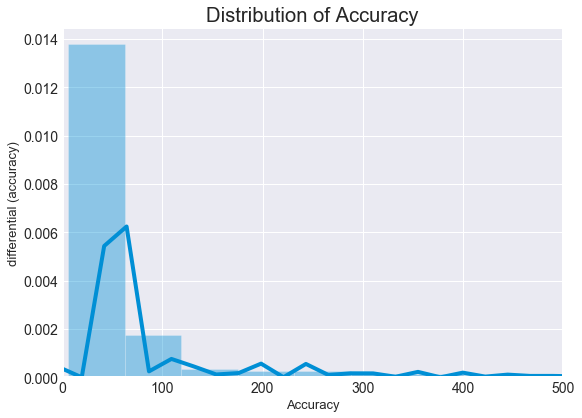Generally environmental factors such as dense trees, steep hillsides, tall buildings, or even heavy cloud cover can impact the travel of the GPS signal between the gps device and the satellites. Under these conditions data accuracy can decrease. We should discard the data with poor accuracy.

In :
```# Remove the rows with accuracy level > 57 m
# The values out of the third quartile
gps_data= gps_data[gps_data["accuracy"]< 57]

# See the size and other parameters of the dataset after removal
gps_data.info()
gps_data.describe()
```
```<class 'pandas.core.frame.DataFrame'>
DatetimeIndex: 15197 entries, 2015-03-01 07:16:39 to 2015-03-31 21:29:27
Data columns (total 4 columns):
utc_time_sec    15197 non-null int64
accuracy        15197 non-null float64
longitude       15197 non-null float64
latitude        15197 non-null float64
dtypes: float64(3), int64(1)
memory usage: 593.6 KB
```
Out:
utc_time_sec accuracy longitude latitude
count 1.519700e+04 15197.000000 15197.000000 15197.000000
mean 1.426308e+09 32.530246 69.886460 20.508920
std 8.155825e+05 9.308392 0.109061 0.206744
min 1.425191e+09 6.000000 69.745429 20.244196
25% 1.425625e+09 27.000000 69.749820 20.254998
50% 1.426053e+09 30.000000 69.973362 20.659323
75% 1.426947e+09 37.500000 69.979196 20.687379
max 1.427830e+09 56.988998 70.130281 20.929037

As we can see now mean and median of the accuracy column is very close to each other

## Feature Creation¶

In this part, two new features are created:

• `time_difference_sec`: Time difference in seconds between two succesive data points
• `distance_km`: Distance in kilometers between two succesive location

### Time intervals are not uniform¶

Let's first look at the time intervals. We observe that the time difference between successive data points are not equal. To see the time differences between successive data points we create a new column which shows the the differences in seconds: `time_difference_sec`

In :
```# Add a column showing the time differences between two successive data points in seconds
gps_data["time_difference_sec"]=gps_data["utc_time_sec"].diff()

# Print the details of time_difference column with .describe() method
print(gps_data.time_difference_sec.describe())

```
```count     15196.000000
mean        173.701500
std        2677.978126
min           2.000000
25%          28.000000
50%          31.000000
75%          61.000000
max      140589.000000
Name: time_difference_sec, dtype: float64
```
Out:
utc_time_sec accuracy longitude latitude time_difference_sec
timestamp
2015-03-01 07:16:39 1425190599 10.0 69.979205 20.687428 NaN
2015-03-01 07:17:12 1425190632 29.0 69.979196 20.687460 33.0
2015-03-01 07:17:25 1425190645 33.0 69.979229 20.687334 13.0

Since the number of intervals between n succesive points is n-1 the first value of the `time_difference_sec` is `"NaN"`

### Time differences on chart¶

Let's see the distribution of the time intervals between two successive data records

In :
```fig, ax=plt.subplots(figsize=(8,6))
gps_data.time_difference_sec.plot(marker="o", linewidth=0)

ax.set_xlabel("Week", fontsize=13)
ax.set_ylabel("Time difference in seconds", fontsize=13)
ax.set_title("Time difference between two subsequent data sample");
```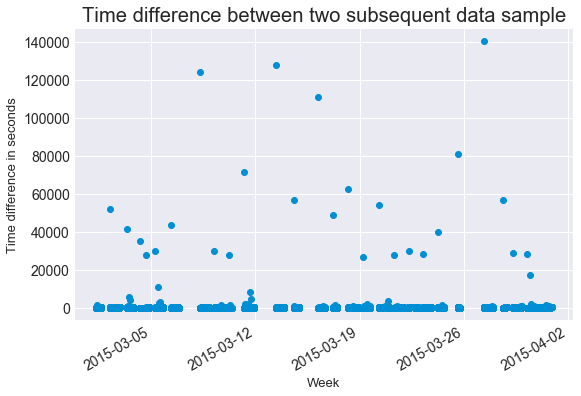As we see on the chart there are some extreme time gaps. This happens due to the connection lost. During this gaps either the device is turned off or gps signal is lost due to the environmental factors.

### Distribution of time gaps¶

In :
```fig, ax=plt.subplots(figsize=(8,4))
sns.distplot(gps_data["time_difference_sec"].dropna(),
rug=True,
rug_kws={"color":"black"})

ax.set_xlabel("Time difference in seconds", fontsize=13)
ax.set_ylabel("d (time difference)", fontsize=13);
ax.set_title("Distribution of Time differences");
#ax.set_xlim(0, 4000);
```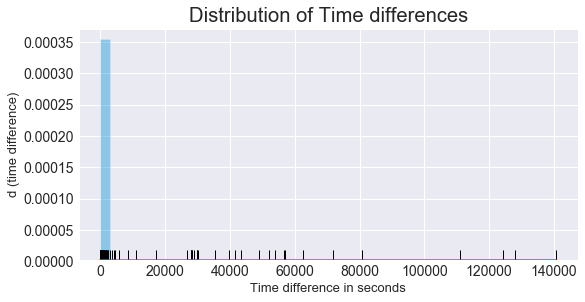### Plot of the coordinates of gps_data¶

It is time to plot the coordinates, latitude and longitude values and see how it is scattered during all the period.

In :
```# Display the joint distribution of longitudes and lattitudes
g= sns.JointGrid(x="longitude",
y= "latitude",
data=gps_data,
height=7)

g= g.plot_joint(sns.kdeplot, cmap="Blues_d")
g= g.plot(sns.regplot, sns.distplot)
```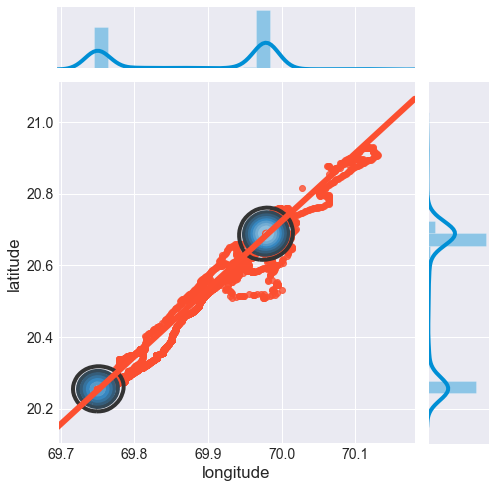We can clearly see from the plot that they are concentrated in two points. The basic idea here is that the user of the device spends her/his time mainly in two spots during the data period. Most likely, one of the two points is the house and the other is the work place. We will try to look in the details of this.

### Distances: Euclidean vs Spherical¶Since the earth is spherical the distance between two cordinates on the surfcace is different than Euclidean distance, as seen in the picture. To calculate the distance from the latitude and longitute there are several methods like Haversine or Vincenty or the other methods. We can use geopy.distance function here.

In :
```# See how the distance function works

# Two coordinate samples with 0.1 change in lattitudes
coords1 = (20.0, 70)
coords2 = (20.1, 70)

# Pass the coordinates to the distance function and
# use the `.km` attribute to get the distance in kilometer
print("When the other component is constant")
print("0.1 change in latitude corresponds", geopy.distance.distance(coords1, coords2).km, " km change")

# Another coordinate samples with 0.1 change in longitude
coords2 = (20, 70)
coords3 = (20, 70.1)

print( "0.1 change in longitude corresponds", geopy.distance.distance(coords2, coords3).km, "km change")
```
```When the other component is constant
0.1 change in latitude corresponds 11.070491302727412  km change
0.1 change in longitude corresponds 10.46470847561311 km change
```

### Subsequent Distances¶

Having seen how the `distance` function works we can use it to get the distances between two subsequent data points.

In :
```# Represent a location as a latitude and longitude tuple and
# create the locations list whose elements are the tuples consist of latitude and longitude values
locations=list(zip(gps_data.latitude, gps_data.longitude))

# Make a list of succesive distances in kms
# by using list comprehensive method and geopy.distance.distance function
distance_lst=[geopy.distance.distance(locations[i-1], locations[i]).km for i in range(1, len(locations))]

# Print the first 3 elements of the distance_lst
distance_lst[:3]
```
Out:
`[0.003621872383733491, 0.014308385103896632, 0.014267680909438382]`

#### Create the `distance_km` column¶

We have created a list of distances covered between two data records. Now we add a distance column to our dataframe

In :
```# Since the number of intervals between n succesive points is n-1
# We need to insert a 0 (zero) in the first position of the list in order to
# match the index size of the gps_data dataframe
distance_lst.insert(0, 0)

# Convert the distance list to an array
distance_ary=np.array(distance_lst)

# Round the distance values to 4 decimals
distance_ary=np.round(distance_ary, 4)

# Add a new column of distances in kms: distance_km
gps_data["distance_km"]= distance_ary

# See the head of the new data frame
```
Out:
utc_time_sec accuracy longitude latitude time_difference_sec distance_km
timestamp
2015-03-01 07:16:39 1425190599 10.0 69.979205 20.687428 NaN 0.0000
2015-03-01 07:17:12 1425190632 29.0 69.979196 20.687460 33.0 0.0036
2015-03-01 07:17:25 1425190645 33.0 69.979229 20.687334 13.0 0.0143

## Grouping for different time frames¶

Time series data can give us ideas about the patterns in different time frames.

We can observe the patterns

• by extracting the time of interest from the timestamp and
• by grouping of the relevant metric column(s) by the time element and
• applying an aggregation method

In this section we try to get some inference by groupping the data in different time frames.
We start from the largest time frame and afterwards analyze the shorter ones.

Since our gps dataset's entire time interval is one month, we first start working on daily data groups and than continue with hourly data groups.

### Splitting the data¶

Before going deeper in grouping, it would be a good practice to split the data into two part as weekdays and weekend.

We know that in general for many people the habits, schedules etc are quite different in these two periods.
For instance, assume that we wake up around 6am during weekdays and around 9am at weekends (bimodal), without splitting the data we can calculate the average wakeup time as aproximately 7am. However this is not a good interpretation because we never wake up at 7am during all the period.

If data contains different patterns in different time frames observing data in separatly give us more robust results.

So let's first split our data as `weekdays` and `weekend`

In :
```# Excrat the weekdays from the gps_data: weekdays
weekdays=gps_data[gps_data.index.weekday < 5]

# Excrat the weekends from the gps_data: weekend
weekend=gps_data[gps_data.index.weekday >= 5]
```

## Plotting Functions¶

In this project we do ploting repeatedly so it would be more efficient to define ploting functions instead of writing the scripts again each time

### `bar_plot` function¶

In :
```# Define the barplot function: bar_plot
def bar_plot(df, label_x, label_y, str_title):
'''Takes a dataframe and three strings: label of x-axis, label of y-axis and title
returns a bar plot'''
plt.style.use('fivethirtyeight')
fig, ax=plt.subplots()
ax= df.plot(kind= "bar",
figsize=(10,5),
rot=70,
fontsize=11)
ax.set_xlabel(label_x)
ax.set_ylabel(label_y)
ax.set_title(str_title)
plt.show()
```

### Daily `number of data records`¶

Having splitted the data into different time frames, now we can look into daily data recording patterns.
Let's count the number of data records in weekdays and weekends.

In :
```# Count the daily data records in weekdays: daily_count
# Groupby the gps_data daily by extracting the "day" from the datetime index and
# count any of the column (without NaN values)
daily_weekdays_count= gps_data.groupby(gps_data.index.dayofweek).accuracy.count()

# Set the index with the names of days
daily_weekdays_count.index=['Mon', 'Tue', 'Wed', 'Thu', 'Fri', "Sat", "Sun"]

labelx="Day"
labely= "Data counts"
title="Daily data count"
bar_plot(daily_weekdays_count, labelx, labely, title)
```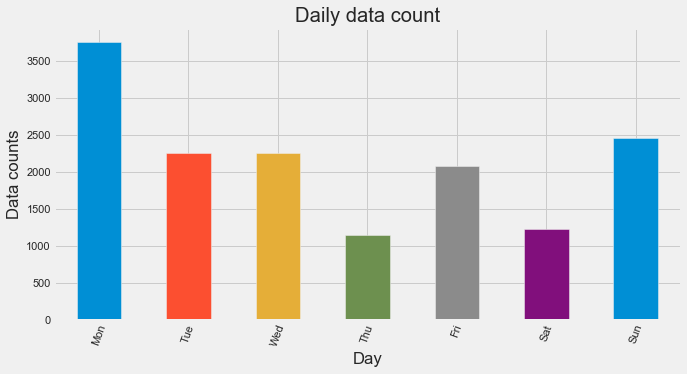### Hourly `number of data records`¶

Observing data recordings based on the hours of the day can give some insight about our dataset.
Now our time frame is 24 hours. Each bar represents an hour of the days so it is better to use splitted datasets.
Here we start by weekdays

In :
```# Count the hourly data records in weekdays: hourly_weekdays_count
# Groupby the weekdays hourly by extracting the "hour" from the datetime index and
# count any of the column (without NaN values)
hourly_weekdays_count= weekdays.groupby(weekdays.index.hour).accuracy.count()

# Set the index with the keys of the group object, here hours
hourly_weekdays_count.index= hourly_weekdays_count.keys()

labelx="Hour"
labely= "Data counts"
title="Weekdays hourly data count"
bar_plot(hourly_weekdays_count, labelx, labely, title)
```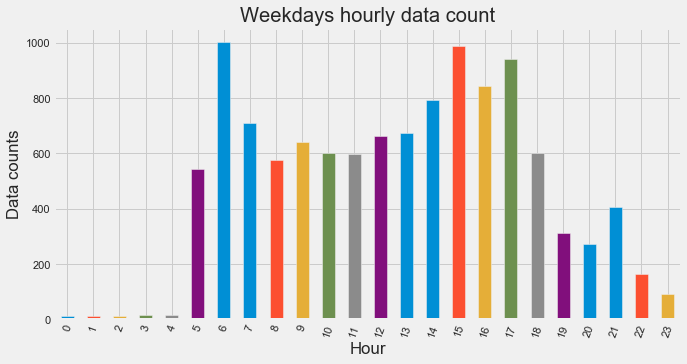In the above chart, to calculate one of the bars, say 10am bar, we sum all the data records of the `weekdays` between `10-11 am during all the weekdays` (excluding weekends).

Lets try to interpret the chart.

• At fist glance it is evident that during the night especially between 0-5 am, we could not get much data.
• Also after 19h the number of data records decreasing.
• This can be related with the device usage habits.
• Maybe the user sometimes turn off the device after 19h and generally after midnight during sleep hours. Or this can be an issue of signal lost that is related with the place where the user spends the time after 19, most likely the home.

Now we plot the same chart for the weekends.

In :
```# Count the hourly data records in weekends: hourly_weekends_count
# Groupby the weekends hourly by extracting the "hour" from the datetime index and
# count any of the column (without NaN values)
hourly_weekends_count= weekend.groupby(weekend.index.hour).accuracy.count()

# Set the index with the keys of the group object, here hours
hourly_weekends_count.index= hourly_weekends_count.keys()

labelx="Hour"
labely= "Data counts"
title="Weekends hourly data count"
bar_plot(hourly_weekdays_count, labelx, labely, title)
```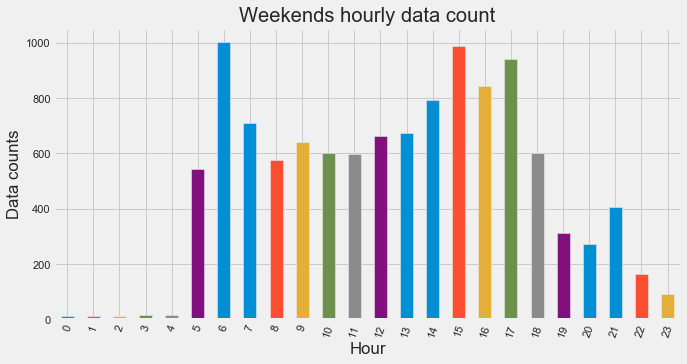We can observe a slight difference between weekdays and weeekend chart especially in the early morning and late night parts

At this point we can process `weekdays` partially in different intervals like `morning, daytime, evening` in order to get additional information about the data records in shorter time frames.

We will do this just for the weekdays data in order to keep this notebook shorter but it can be easily applied on the weekend data in the same way as weekdays.

### Number of data recording days¶

In :
```# Slice the period 0-5 hours from the weekdays df: night_weekdays
night_weekdays=weekdays[(weekdays.index.hour>=0) & (weekdays.index.hour<5)]

# Days in which we get data during 0-5 hours
# Extract the unique days by .unique() method
days0_5=night_weekdays.index.day.unique()

# Print all the weekdays first
print('All the weekdays of the dataset:', "\n", f'{set(weekdays.index.day)}')
print(f'({len(set(weekdays.index.day))} days)', "\n")

# Print only the days we record data
print("Data recording days during 0h-5h:", "\n", list(days0_5))
```
```All the weekdays of the dataset:
{2, 3, 4, 5, 6, 9, 10, 11, 13, 16, 17, 18, 19, 20, 23, 24, 25, 27, 30, 31}
(20 days)

Data recording days during 0h-5h:

```

The result tells us that we could get data only for one day, 31st day. Now same process for the daytimes

In :
```# Slice the period 5-19 hours from the weekdays df: night_weekdays
daytime_weekdays=weekdays[(weekdays.index.hour>=5) & (weekdays.index.hour<19)]

# Days in which we get data during 5-19 hours
days5_19= daytime_weekdays.index.day.unique()

# Print only the days we record data
print("Data recording days during 5h-19h:", "\n", list(days5_19))
print(f'({len(list(days5_19))} days)')
```
```Data recording days during 5h-19h:
[2, 3, 4, 5, 6, 9, 10, 11, 13, 16, 17, 18, 19, 20, 23, 24, 25, 27, 30, 31]
(20 days)
```

We have data for all the weekdays daytime. Same process for the evening period

In :
```# Slice the period 19-24 hours from the weekdays df: night_weekdays
evening_weekdays=weekdays[(weekdays.index.hour>=19) & (weekdays.index.hour<24)]

# Days in which we get data during 19_24 hours
days19_24= evening_weekdays.index.day.unique()

# Print only the days data is recorded
print("Data recording days during 19_24:", "\n", list(days19_24))
print(f'({len(list(days19_24))} days)')
```
```Data recording days during 19_24:
[2, 3, 4, 5, 6, 9, 11, 13, 18, 20, 27, 30, 31]
(13 days)
```

The numbers of data receiving days better explains the "Weekdays hourly data count" chart.

## `Movement` in weekdays¶

After data count, we can look for the movement patterns.
We are interested in how the user changes places.
Is there any pattern in his/her movements?

As you know we calculated the distance between two positions in two subsequent data points and created the `distance_km` column.

Here our approach is

• to sum the distances to find the total movement in a time frame and
• divide it into the number of the number of that time frame to find the average movent.

As usual we from the larger time frame i.e daily

### `Average Movement` based on the `days of the week`¶

In :
```# Find the average daily distance_km values in weekdays df: daily_movement
# Groupby the the distance_km column values daily and aggregate by mean
daily_movement=weekdays.groupby(weekdays.index.dayofweek).distance_km.mean()

# Set the index by the names of the weekdays
daily_movement.index = ['Mon', 'Tue', 'Wed', 'Thu', 'Fri']

# Plot the chart
labelx="Day"
labely= "Total movement (km)"
title="Weekdays daily movement"
bar_plot(daily_movement, labelx, labely, title)
```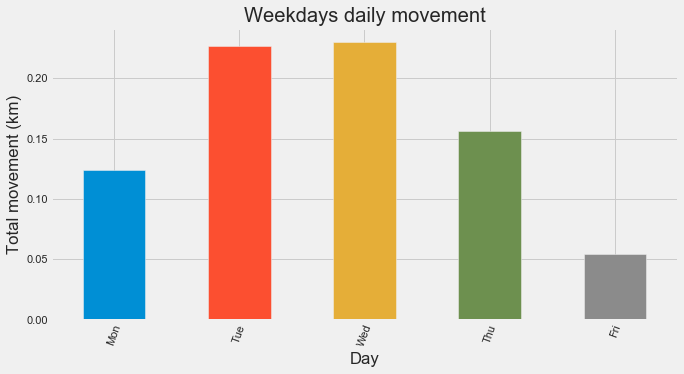In the chart above, to calculate one of the bars, say Tue bar,

• we sum all the distance changes of the user during all the tuesdays and
• divide it into the number of all the tuesdays over all the dataset.

It is easily seen that on fridays the user changes place significantly less than other weekdays.
What would be the reason?

Let's plot the coordinates based on a day of the week to see if there is any answer for less movement on fridays.

### Define a plot function: `group_plot_coord`¶

First we need to define a plot function to plot the coordinates of shorter time frames subsequently so whenever we need to plot subplots, instead of writing the scripts again we can call the function.

Here is the steps:

• First we group the dataframe by the relevant time frame then
• plot the coordinates of each group on the entire coordinates of the dataset
• by iterating over the groupby object
In :
```def group_plot_coord(groupby_obj, title_func):
'''Takes a groupby object and a function (to create the titles of the subplots)
returns a subplot for each of the group'''

# Use the 'fivethirtyeight' style
plt.style.context('fivethirtyeight')
# associate the row number argument in plt.subplots with groupby obj
row_number=int(len(groupby_obj)/2)+1

# Create figure and axes
# We fix the number of column of the figure by ncols=2 and
# Let the row_number change by the size of the groupby object
# We will delete the last empty subplots at the end
fig, axes = plt.subplots(nrows=row_number, ncols=2,
figsize=(16, 16),
sharex=True,
sharey=True)

# Flatten the axes and convert into a list in order to be able to pop the elements one by one
axes_lst=list(axes.flatten())

# Iterate over the groups in the groupby object
for group_name, group in groupby_obj:
# Assign the first ax in the ax_lst as current ax
ax=axes_lst.pop(0)
# Create the scatter plot of latitudes and longitudes of entire gps_data df
ax.scatter(gps_data["longitude"], gps_data["latitude"],
color=".5",
edgecolor="white",
s=50)
# Plot the lattidues and longitudes of each group on the same ax
group.plot(x="longitude", y="latitude",
ax=ax,
kind="scatter",
legend=True,
label=group_name,
s=60,
c="r",
edgecolor="white")
# Set the title of each subplot
ax.set_title(title_func(group_name))
plt.show()

# Use matplotlib .remove() method to delete the last empty subplot
[ax.remove() for ax in axes_lst];
```

### Coordinates plot based on the weekdays¶

• First we group the `weekdays` dataframe by days of week
• Then we iterate over the groups, in this case the days and
• plot the coordinates of each day over the coordinates of entire dataset
In :
```# Groupby the weekdays dataframe based on days of the week: daily_group
daily_groups=weekdays.groupby(weekdays.index.dayofweek)

# Create a dictionary of day names in order to use for the titles of subplots
day_dict={0:"Monday", 1:"Tuesday", 2:"Wednesday", 3:"Thursday", 4:"Friday"}

# define the title func:title_func
def daily_title(name):
return day_dict[name]
# Plot the coordinates of all the weekdays
group_plot_coord(daily_groups, daily_title)
```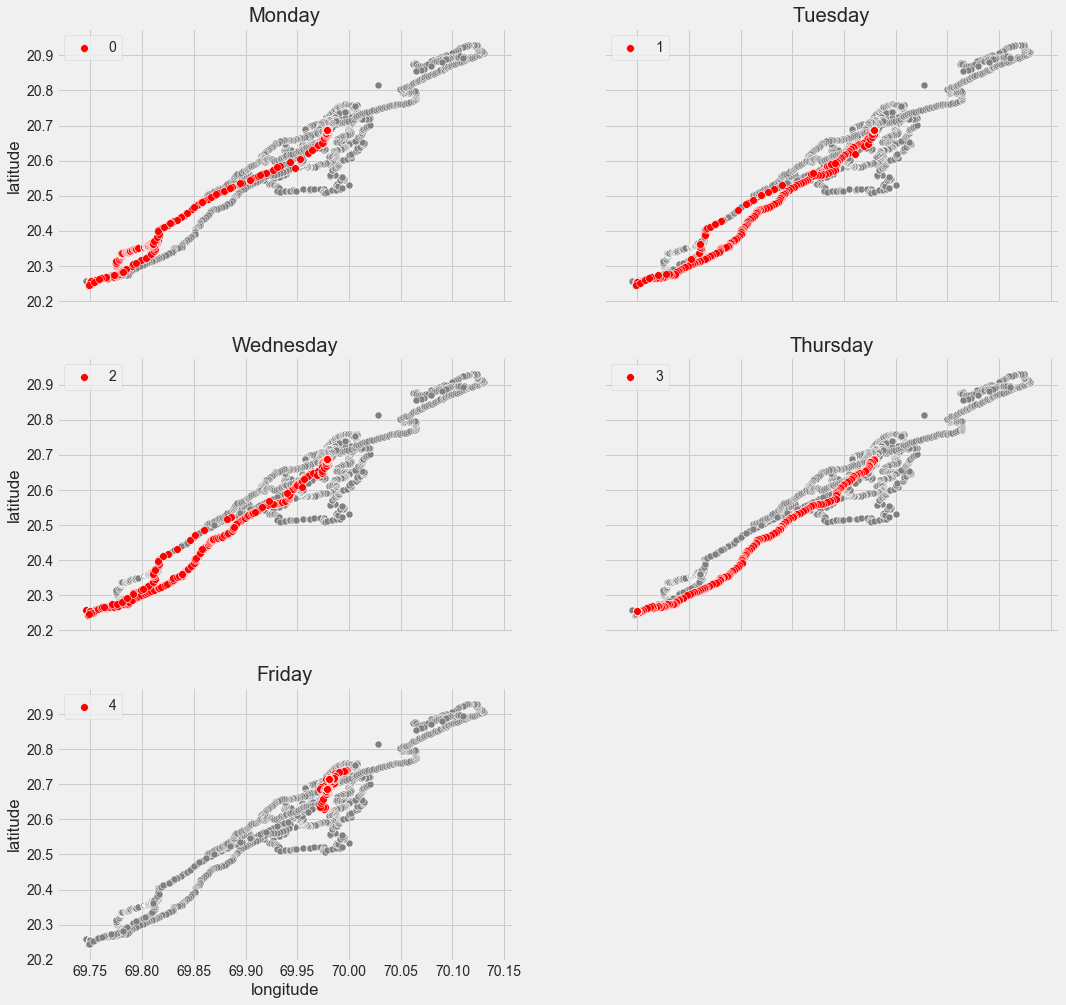In the above charts daily subplots give us some more information about the coordinates distribution during the weekdays.
Here we notice that the movement on friday is different than the other days. On fridays the user does not follow a track like the other weekdays.

We can make a guess that the user going to work monday to thursday regularly but on fridays he/she works from home or another.
We can also think that it is an off day but the coordinates are not dispersed so the user stay around one spot most likely the home

Now lets also look at the distribution of the daily coordinates.

Like we did with other charts first we need to define a distribution function for reusebility.

### Define a joint distribution plot function: `full_joint_plot`¶

In :
```# Display the joint distribution of longitudes and lattitudes
def full_joint_plot(df, title_str):
'''Takes a dataframe and title string and returns a joint distribution of the coordinates'''
sns.axes_style("darkgrid")
g= sns.JointGrid(x="longitude",
y= "latitude",
data=df,
height=7)
g.plot_joint(sns.kdeplot, cmap="Blues_d")
sns.kdeplot(df["longitude"], ax=g.ax_marg_x, legend=False)
sns.kdeplot(df["latitude"], ax=g.ax_marg_y, vertical=True, legend=False)
g.ax_joint.plot(df["longitude"], df["latitude"], "o", ms=5)
g.fig.suptitle(title_str, x=0.4, y=0.81);
```

### Coordinates distribution of `monday-to_thursday`¶

Since we noticed that the movement pattern is different on fridays than the days from monday to thursday, it is better to visualize the distributions separately.

Let's first look at the distribution of the `monday_to_fridays`

In :
```# Slice the rows of the day monday-to-thursday from gps_data: mon_to_thu
mon_to_thu= gps_data[gps_data.index.dayofweek < 4]
title_str="Coordinates distribution monday-to-thursday"
full_joint_plot(mon_to_thu, title_str)
```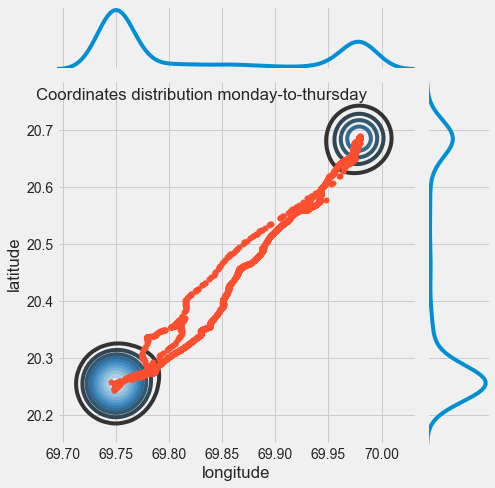### Coordinates distribution of `fridays`¶

In :
```# Slice the rows on fridays from gps_data: fridays
fridays=gps_data[gps_data.index.dayofweek==4]

title_str="Coordinate distribution on fridays"
full_joint_plot(fridays, title_str)
```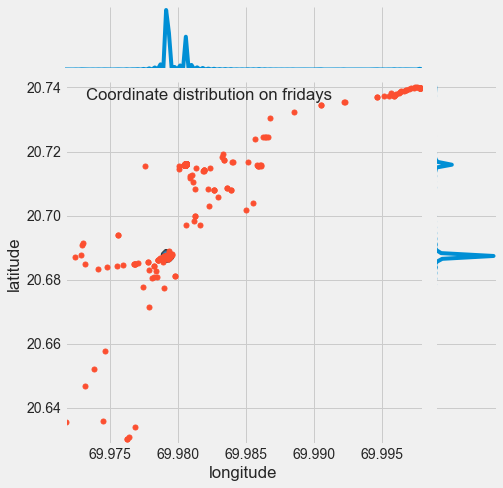Now we can continue our analyse of movement in the hourly time frame.

### `Average movement` based on the `hours of the days`¶

Here we try to stick to our approach and visualize the hourly average moment seperately for monday-to-thursday and for fridays periods.

##### Monday to thursday hourly movement¶
In :
```# Find the average hourly distance_km values in mon_to_thu df: hourly_movement1_4
# Groupby the the distance_km column values hourly and aggregate by mean
hourly_movement1_4=mon_to_thu.groupby(mon_to_thu.index.hour).distance_km.mean()

# Set the index by the keys of the group object, here hours
hourly_movement1_4.index = hourly_movement1_4.keys()

# Plot the chart
labelx="Hour"
labely= "Average movement (km)"
title="Monday-to-thursday hourly movement"
bar_plot(hourly_movement1_4, labelx, labely, title)
```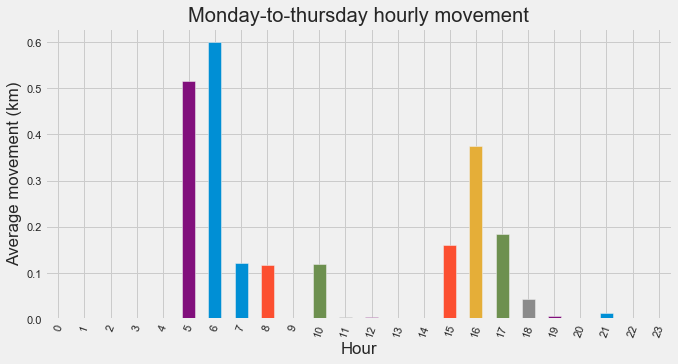##### Fridays hourly movement¶
In :
```# drop the outlier
fridays=fridays.drop(pd.to_datetime("2015-03-20 07:21:58"))
```
In :
```# Find the average hourly distance_km values in fridays df: hourly_movement4
# Groupby the the distance_km column values hourly and aggregate by mean
hourly_movement4=fridays.groupby(fridays.index.hour).distance_km.mean()

# Set the index by the keys of the group object, here hours
hourly_movement4.index = hourly_movement4.keys()

# Plot the chart
labelx="Hour"
labely= "Average movement (km)"
title="Fridays hourly movement"
bar_plot(hourly_movement4, labelx, labely, title)
```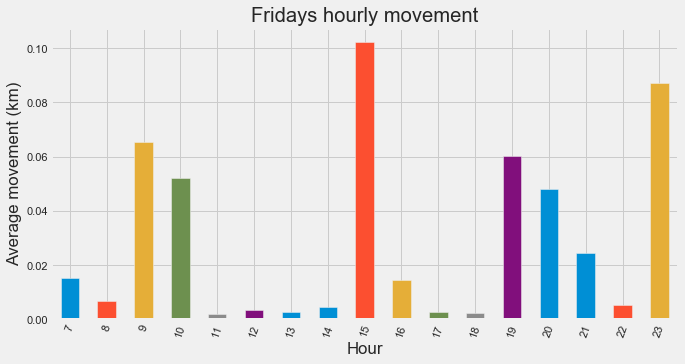### Hourly coordinates plot¶

To visualize the hourly movements lets plot the coordinates in hourly time frames.

But first we need to split the `mon_to_thu` and `fridays` dataframes into 2: morning, afternoon.

In :
```# Slice the morning times from mon_to_thu df
morning0_3=mon_to_thu[(mon_to_thu.index.hour>=5)& (mon_to_thu.index.hour<12)]

# Groupby the morning_weekdays hourly: hourly_morning
hourly_morning0_3=morning0_3.groupby(morning0_3.index.hour)

def hourly_title(name):
return f'Coordinates during {name}h-{name+1}h'

group_plot_coord(hourly_morning0_3, hourly_title)
```